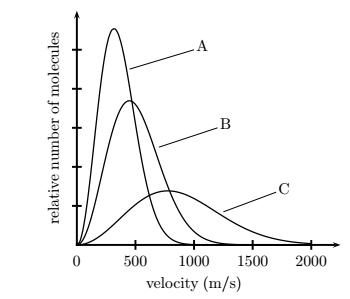# Problem: The graph shows the Maxwell distribution plots for a given gas at three different temperatures. Which plot corresponds to the highest temperature?1. B2. Cannot be determined from this type of plot.3. C4. A

92% (96 ratings)
###### Problem Details

The graph shows the Maxwell distribution plots for a given gas at three different temperatures. Which plot corresponds to the highest temperature?

1. B

2. Cannot be determined from this type of plot.

3. C

4. AWhat scientific concept do you need to know in order to solve this problem?

Our tutors have indicated that to solve this problem you will need to apply the Velocity Distributions concept. You can view video lessons to learn Velocity Distributions. Or if you need more Velocity Distributions practice, you can also practice Velocity Distributions practice problems.

What is the difficulty of this problem?

Our tutors rated the difficulty ofThe graph shows the Maxwell distribution plots for a given g...as medium difficulty.

How long does this problem take to solve?

Our expert Chemistry tutor, Sabrina took 2 minutes and 4 seconds to solve this problem. You can follow their steps in the video explanation above.

What professor is this problem relevant for?

Based on our data, we think this problem is relevant for Professor Smith's class at UH.